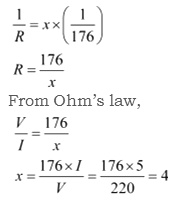3 Tutor System
Starting just at 265/hour

# How many 176 $$\Omega$$ resistors (in parallel) are required to carry 5 A on a 220 V line?

For x number of resistors of resistance 176 $$\Omega$$, the equivalent resistance of the resistors connected in parallel is given by Ohm's law as
V= IR R= $$\frac{V}{I}$$ Where, Supply voltage, V= 220 V Current, I = 5 A Equivalent resistance of the combination = R,given asTherefore, four resistors of 176 $$\Omega$$ are required to draw the given amount of current.